# NumS: NumPy API-Compatible Framework backed by Ray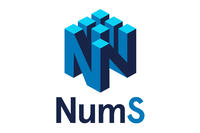Runtime improvements to nd-array and tensor operations increasingly rely on parallelism, and the scientific computing community has a growing need to train on more data. Systems and machine learning research has focused mostly on scaling machine learning workloads by designing scalable solutions for specific machine learning problems, such as data parallel optimization algorithms. However, using these solutions typically requires new tooling and knowledge of networking within and between various operating systems, making them less accessible to Python programmers already familiar with existing scientific computing tools that do not require such systems knowledge. As an alternative to scaling specific algorithms, a more general solution to these problems can be achieved through a high-performance scalable implementation of the NumPy API, the ubiquitous Python-based API for nd-array and linear algebra programming. In this work, we present a novel approach to optimizing NumPy programs at runtime while maintaining an array abstraction that is faithful to the NumPy API, bridging the knowledge gap required to obtain good performance on clusters of computational resources for common machine learning workloads. Our implementation, called NumS, is able to provide up to 10x speedup on basic linear algebra operations and up to 6x speedup on logistic regression compared to Dask, a popular distributed system which provides a NumPy-like programming API.

### Researchers

September 1st, 2021

### Overview

#### Design

NumS is an eager evaluation distributed array library, which allows for efficient and seamless integration with branch and loop commands in Python. The NumS BlockArray is a mutable data structure, just like NumPy arrays. The NumS optimizer eliminates the need to learn a new programming model to achieve data (and model) parallel training. These are carefully made decisions that maximize both performance and usability.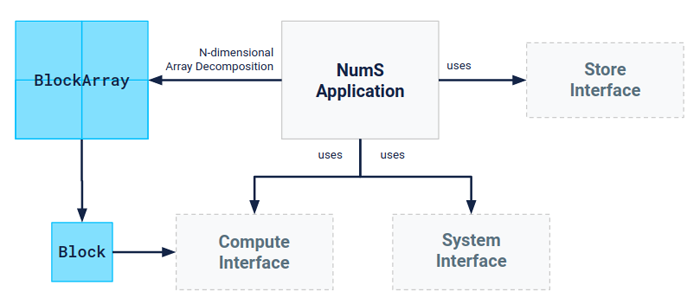NumS implements nd-arrays as futures, and operations are carried out as promises. This captures exactly the data dependencies required to compute each block in the output array, allowing for concurrent execution of independentoperations within and between output blocks. Whenever an array access is triggered, high-level operations on the resulting new array are captured as low-level block operations. This implicitly constructs a computation graph, which is leveraged by NumS’s distributed system implementation to concurrently execute independent operations.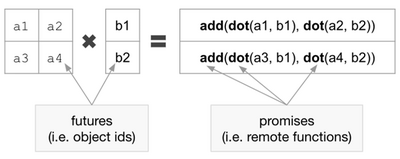Like NumPy arrays, the NumS BlockArray has a shape, but it also requires a block_shape in order to organize the contents of arrays into discrete blocks which can be distributed across multiple memory devices. The block_shape each blocks shape. A BlockArray also has a grid_shape, which determines the layout of a BlockArray over a collection of memory devices.

#### Block-Cyclic Optimizer

To achieve acceptable performance on distributed memory devices, NumS must decide on which devices to operate on and store data. Each Block in a BlockArray has associated with it a grid_entry, which indicates where within the grid structure of the BlockArray the Block is stored. Similarly, a node cluster is represented as a grid of nodes, where the cluster has a grid_shape and each node has a grid_entry. To achieve block-cyclic scheduling, blocks and operations on blocks are dispersed in the cluster by selecting a node using the mod operation, wrapping to the beginning of a cluster grid axis whenever the end of that axis is reached. To take advantage of the benefits of this approach while mitigating its shortcomings, we construct arrays of computation trees that we optimize using our optimizer, and output the results of the optimization procedure according to the block-cyclic scheduling heuristic. Our optimizer consists of the following components:

• Objective: The overall objective of the optimizer is to place operations so that maximum memory and network load over all nodes is minimized.
• Cluster State: The optimizer maintains an estimate of the cluster state, which contains memory and network load on each node.
• Computation State: The optimizer progresses the computation of a computation graph by iteratively updating an array-of-trees, called the GraphArray.
• TreeSearch: The optimizer is a tree search algorithm, which places a single operation per iteration according to the objective, and updates both the cluster state and computation state.

Below is an example of element-wise addition, illustrating the transition from the BlockArray representation to the GraphArray representation, which is used to optimize computations at runtime.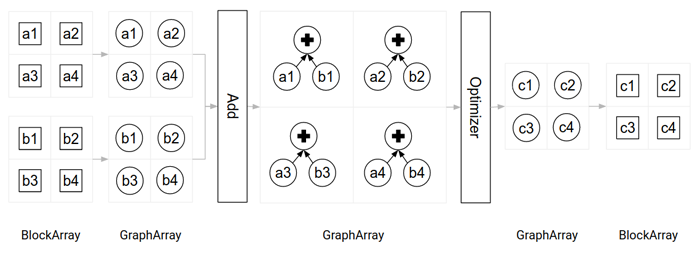#### Results

We benchmark the execution time of loading, executing, and evaluating the correctness of various operations, QR-decomposition, and logistic regression. A missing result means we were unable to perform the operation because Dask ran out of memory. Each node has 64 cores, 512 RAM over 75Gbps network. All data read in from S3. Ray/Dask with 64 workers-per-node. NumS configured to use (16, 1) cluster shape.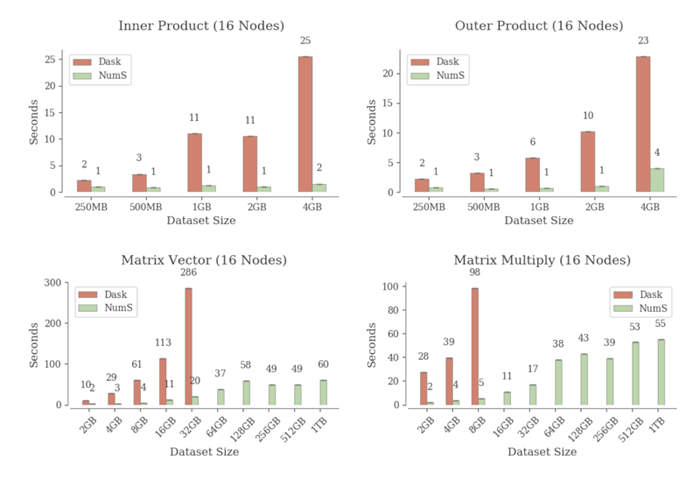Our performance on basic operations can be largely attributed to the combination of a tall-skinny cluster shape combined with our optimizer. We place objects initially using block-cyclic scheduling, and use our optimizer to perform the actual operations. These are at most pairwise products followed by sum reductions.

#### QR Decomposition and Logistic RegressionBoth QR-decompositions execute the direct tall-skinny QR decomposition algorithm. Dask's implementation performs better under resource constraints, but we believe this is due to a difference in algorithm implementation (not an inherent flaw in the design of NumS).

Logistic regression is performed on a bimodal Gaussian, optimized using Newton’s method. Our preliminary analysis suggests our optimizer performs data-parallel training per iteration of Newton’s method. We're currently investigating how close our optimizer gets to a communication optimal reduction when averaging distributed gradients.

### Appendix

Operations and memory layout of input data:

• Inner product
• op = y.T @ y
• grid = (64, 1)
• Outer product
• op = y @ y.T
• grid = (64, 1)
• Matrix multiplication
• op = X.T @ X
• grid = (1024, 1) for 1TB
• Matrix-vector
• op = X.T @ y
• grid = (1024, 1) for 1TB
• QR-decomposition
• grid = (1024, 1) for 1TB
• Logistic Regression
• grid = (1024, 1) for 1TB